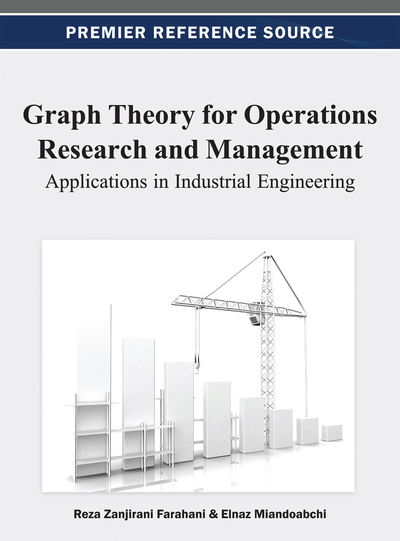# Networks

Alireza Boloori (Wayne State University, USA) and Monirehalsadat Mahmoudi (Amirkabir University of Technology, Iran)
DOI: 10.4018/978-1-4666-2661-4.ch012

## Abstract

This chapter sheds light on various aspects of network theory and relevant concepts including basic definitions, the most common types of network flow problems that have been studied by literature, and the main algorithms and solution approaches proposed for solving network problems.
Chapter Preview
Top

## Network Flow Theory

In this part, some fundamental definitions and elements of the theory of network flows are presented which set an essential framework in the analysis of network.

As many references explained (see Bang-Jensen & Gutin, 2007; Bondy & Murty, 2008; Cormen et al., 2009; Diestel, 2005; Gross & Yellen, 2003; West, 2001; Xu, 2003), if V and E are respectively considered as the sets of vertices and edges in a directed graph, then G=(V,E) will be a network in which each edge (i,j) (i and j are two consecutive vertices) is restricted by a lower bound l(i,j) and an upper bound u(i,j). l(i,j) is usually set for some types of networks, in which the minimum amount of flow is required. For example, due to the importance of pipeline networks conveying oil from refinery centers to main cities, the flow along each edge must be at least equal to its lower bound so that any shortage will not happen in the oil transmission. On the other hand, u(i,j) is generally called the capacity c(i,j) of that edge, by which the flow f(i,j) for each edge cannot exceed its capacity. Specifically, the following condition can be considered for f(i,j): l(i,jf(i,ju(i,j). Concerning this definition, it should be noted that V and E might denote the number of vertices and edges in a network as well.

## Complete Chapter List

Search this Book:
Reset